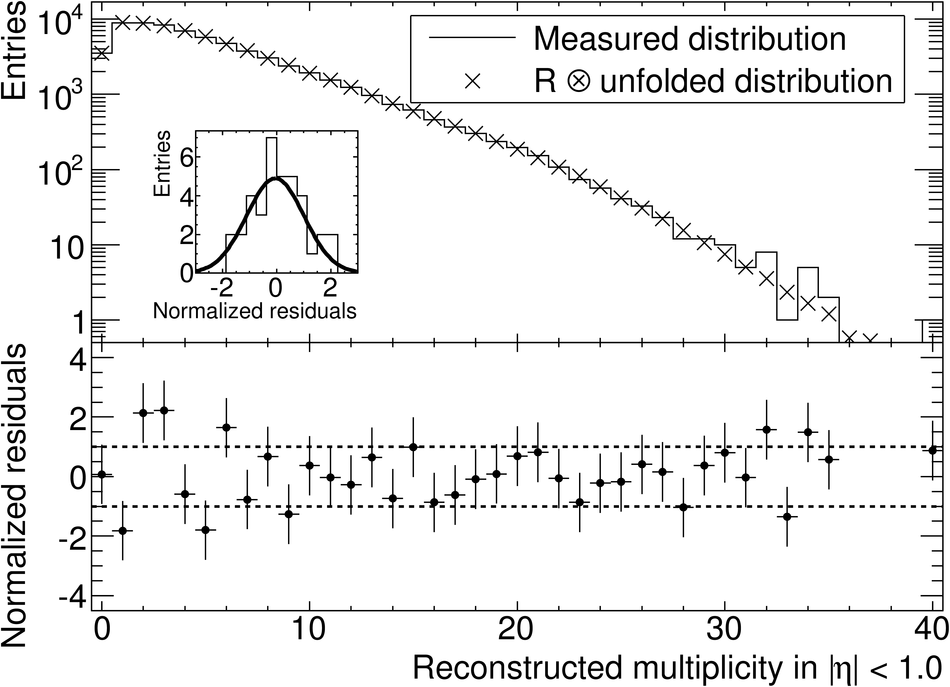# Figure 5

 Measured raw multiplicity distribution (elements of vector $M$, histogram), superimposed on the convolution $RU$ of the unfolded distribution with the response matrix (crosses), at $\sqrt{s} = 0.9~$TeV for $|\eta| < 1.0$ (upper plot). The error bars are omitted for visibility Normalized residuals, i.e. the difference between the measured raw distribution and the corrected distribution folded with the response matrix divided by the measurement error (lower plot) The inset shows the distribution of these normalized residuals fitted with a Gaussian ($\sigma \approx 1.06$).